## 3.7Laplacian discretisation

Let us ﬁrst describe the discretisation of the Laplacian term for diﬀusion, introduced in Sec. 2.14 .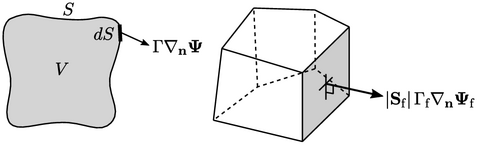Following the ﬁnite volume principles described in Sec. 3.1, the discretisation approximates the surface integral by a summation over faces by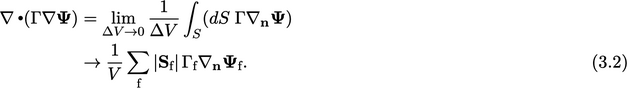The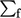in Eq. (3.2 ) can be viewed as the summation over faces of a single cell. Applying the summation to all cells provides the contribution to coeﬃcients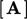and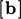of a matrix equation.

The mesh data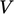and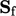are calculated according to Sec. 3.3 so the remaining properties to be determined are:

• the diﬀusivity at faces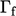;
• the surface normal gradient at faces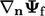.

Fields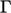and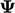are associated with cells, so numerical schemes are required to evaluate properties at faces. We will ﬁrst describe interpolation for, for which the linear scheme is generally used. The surface normal gradient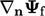is discussed in Sec. 3.8 .

### Interpolation from cells to faces

Mapping data between diﬀerent locations is a common practice in numerics. Since the ﬁnite volume method is concerned with ﬂuxes at faces, the principal mapping procedure is interpolation from cells to faces. Since other interpolations are much less common, it can be assumed that the term “interpolation” means from cell to face unless stated otherwise.

For irregular polyhedral meshes, interpolation is generalised by deﬁning a weights ﬁeld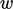for each face according to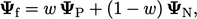(3.3)
where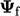is the interpolated face ﬁeld. The subscripts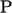and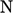indicate values at owner and neighbour cells, respectively.

### Linear interpolation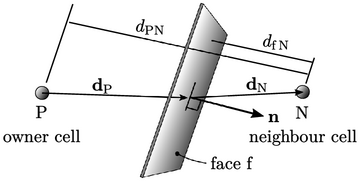The linear interpolation scheme setsaccording to a linear variation between cells values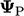and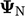. The weights can then be calculated based on distances from the face centre to adjacent cell centres, in the direction normal to the face, by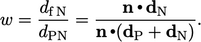(3.4)
Notes on CFD: General Principles - 3.7 Laplacian discretisation